# NCERT Solutions For Class 6 Maths Chapter 10 Mensuration

NCERT Solutions For Class 6 Maths Chapter 10 Mensuration

### NCERT Solutions For Class 6 Maths Chapter 10 Mensuration Ex 10.1

NCERT Solutions For Class 6 Maths Chapter 10 Mensuration Ex 10.1

Exercise 10.1

Ex 10.1 Class 6 Maths Question 1.
Find the perimeter of each of the following figures: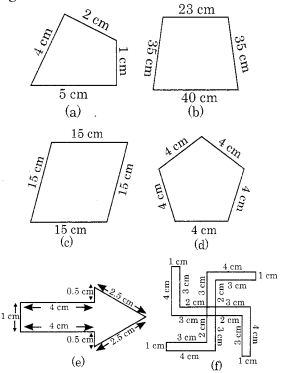Solution:
(a) Required perimeter
= 4 cm + 2 cm + 1 cm + 5 cm = 12 cm
(b) Required perimeter
= 40 cm + 35 cm + 23 cm + 35 cm
= 133 cm or 1.33 m
(c) Required perimeter
= 15 cm + 15 cm + 15 cm + 15 cm = 15 cm x 4 = 60 cm
(d) Required perimeter
= 4 cm + 4 cm + 4 cm + 4 cm + 4 cm – 4 cm x 5 = 20 cm
(e) Required perimeter
= 4 cm + 0.5 cm + 2.5 cm + 2.5 cm + 0.5 cm + 4 cm + 1 cm
= 15 cm
(f) Required perimeter = 4 cm + 1 cm + 3 cm + 2 cm + 3 cm + 4 cm + 1 cm + 3 cm + 2 cm + 3 cm + 4 cm + 1 cm + 3 cm + 2 cm + 3 cm + 4 cm + 1 cm + 3 cm + 2 cm + 3 cm
= 52 cm

Ex 10.1 Class 6 Maths Question 2.
The lid of a rectangular box of sides 40 cm by 10 cm is sealed all round with tape. What is the length of the tape required?
Solution:
Total length of the tape required = perimeter of the rectangular lid
= 2 [length + breadth] = 2 x [40 + 10]
= 2 x 50 = 100 cm

Ex 10.1 Class 6 Maths Question 3.
A table-top measures 2 m 25 cm by 1 m 50 cm. What is the perimeter of the table-top?
Solution:
Length of table-top = 2 m 25 cm
Breadth of table-top = 1 m 50 cm
∴ Perimeter of the table top = 2 [length + breadth]
= 2 [2 m 25 cm + 1 m 50 cm]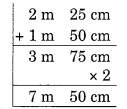= 2 x 3 m 75 cm
= 7 m 50 cm
= 7.5 m

Ex 10.1 Class 6 Maths Question 4.
What is the length of the wooden strip required to frame a photograph of length and breadth 32 cm and 21 cm respectively?
Solution:
Length of the strip = 32 cm
Breadth of the strip = 21 cm
∴ Perimeter = 2 [length + breadth]
= 2 [32 cm + 21 cm]
= 2 x 53 cm = 106 cm
Hence, the required length of the strip = 106 cm or 1 m 6 cm.

Ex 10.1 Class 6 Maths Question 5.
A rectangular piece of land measures 0.7 km by 0.5 km. Each side is to be fenced with 4 rows of wires. What is the length of the wire needed?
Solution:
Length of the rectangular piece of land = 0.7 km = 0.7 x 1000 m = 700 m
Breadth of the rectangular piece of land = 0.5 km = 0.5 x 1000 m = 500 m
∴ Perimeter of the rectangular land
= 2 [700 m + 500 m]
= 2400 m.
Length of wire needed in 4 rounds of the land = 4 x 2400 = 9600 m = 9.6 km.

Ex 10.1 Class 6 Maths Question 6.
Find the perimeter of each of the following shapes:
(a) A triangle of sides 3 cm, 4 cm and 5 cm.
(b) An equilateral triangle of side 9 cm.
(c) An isosceles triangle with equal sides 8 cm each and third side 6 cm.
Solution:
(a) We know that the perimeter of the given triangle = The sum of all sides of the triangle
∴ Perimeter of the triangle = 3 cm + 4 cm + 5 cm = 12 cm
(b) We know that the perimeter of the given triangle
= Sum of all the sides of the triangle
= (9 + 9 + 9) = 27 cm
(c) Perimeter of the given isosceles triangle
= Sum of all the sides of the triangle
= (8 + 8 + 6) cm = 22 cm

Ex 10.1 Class 6 Maths Question 7.
Find the perimeter of a triangle with sides measuring 10 cm, 14 cm and 15 cm.
Solution:
Perimeter of a triangle = Sum of all the sides of the triangle
= 10 cm + 14 cm + 15 cm
= 39 cm

Ex 10.1 Class 6 Maths Question 8.
Find the perimeter of a regular hexagon with each side measuring 8 m.
Solution:
Perimeter of a regular hexagon = 6 x side = 6 x 8 m = 48 m.

Ex 10.1 Class 6 Maths Question 9.
Find the side of the square whose perimeter is 20 m.
Solution:
Perimeter of a square = 4 x side
20 = 4 x side
∴ side = 20 m ÷ 4 = 5 m

Ex 10.1 Class 6 Maths Question 10.
The perimeter of a regular pentagon is 100 cm. How long is its each side?
Solution:
We have
Perimeter of the regular pentagon = 100 cm
Number of sides in regular pentagon = 5
∴ Length of each side = Perimeter ÷ Number of sides
= 100 cm ÷ 5 = 20 cm.

Ex 10.1 Class 6 Maths Question 11.
A piece of string is 30 cm long. What will be the length of each side if the string is used to form:
(a) a square?
(b) an equilateral triangle?
(c) a regular hexagon?
Solution:
(a) Length of string = 30 cm
Number of equal sides in a square = 4
∴ Length of each side of the square = 30 cm ÷ 4 = 7.50 cm.

(b) Length of string = 30 cm
Number of equal sides in equilateral triangle = 3
∴ Length of each side of the equilateral triangle = 30 cm ÷ 3 = 10 cm

(c) Length of string = 30 cm
Number of equal sides in regular hexagon = 6
∴ Length of each side of the regular hexagon = 30 cm ÷ 6 = 5 cm

Ex 10.1 Class 6 Maths Question 12.
Two sides of a triangle are 12 cm and 14 cm. The perimeter of the triangle is 36 cm. What is its third side?
Solution:
Perimeter of the triangle = 36 cm.
Length of two of its sides are 12 cm and 14 cm.
Length of the third side of the triangle = 36 – (12 + 14) cm
= (36 – 26) cm = 10 cm

Ex 10.1 Class 6 Maths Question 13.
Find the cost of fencing a square park of side 250 m at the rate of? 20 per metre.
Solution:
Length of the side of a square = 250 m
∴ Perimeter of the square = 250 m x 4 = 1000 m
Rate of fencing = ₹20 per m.
∴ Cost of fencing = ₹20 x 1000 = ₹20,000

Ex 10.1 Class 6 Maths Question 14.
Find the cost of fencing a rectangular park of length 175 m and breadth 125 m at the rate of ₹12 per metre.
Solution:
Length of the rectangular park = 175 m
Breadth of the rectangular park = 125 m
∴ Perimeter of the park = 2 [length + breadth]
= 2[175 m + 125 m]
= 2 x 300 m = 600 m
Rate of fencing = ₹ 12 per metre Cost of fencing = ₹12 x 600 = ₹7200

Ex 10.1 Class 6 Maths Question 15.
Sweety runs around a square park of side 75 m. Bulbul runs around a rectangular park with length 60 m and breadth 45 m. Who covers less distance?
Solution:
Side of the square park = 75 m
∴ its perimeter = 4 x 75 m = 300 m
Perimeter of the rectangular park = 2 [length + breadth]
= 2 [60 m + 45 m]
= 2 x 105 m = 210 m.
Since 210 m < 300 m.
So, Bulbul covers less distance.

Ex 10.1 Class 6 Maths Question 16.
What is the perimeter of each of the following figures? What do you infer from the answers?Solution:
(a) Perimeter of the square = 25 cm + 25 cm + 25 cm + 25 cm = 4 x 25 cm = 100 cm
(b) Perimeter of the rectangle = 30 cm + 20 cm + 30 cm + 20 cm – 2 [30 cm + 20 cm] = 2 x 50 cm = 100 cm
(c) Perimeter of the rectangle = 40 cm + 10 cm + 40 cm + 10 cm = 2 [40 cm + 10 cm] = 2 x 50 cm = 100 cm
(d) Perimeter of the triangle = Sum of all sides = 30 cm + 30 cm + 40 cm = 100 cm From the above answers, we conclude that different figures may have equal perimeters.

Ex 10.1 Class 6 Maths Question 17.
Avneet buys 9 square paving slabs, each with a side of 1/7 m. He lays them in the form of a square.
(a) What is the perimeter of his arrangement [Fig. (i)]?
(b) Shari does not like his arrangement. She gets him to lay them out like a cross. What is the perimeter of her arrangement [Fig. (ii)]?
(c) Which has greater perimeter?
(d) Avneet wonders, if there is a way of getting an even greater perimeter. Can you find a way of doing this? (The paving slabs must meet along complete edges, i.e., they can not be broken).Solution:
(a) The arrangement is in the form of a square of side(b) Perimeter of cross-arrangement(c) Since 10 m > 6 m
∴ Cross-arrangement has greater perimeter.
(d) Total number of tiles = 9
∴ We have the following arrangementThe above arrangement will also have the greater perimeter.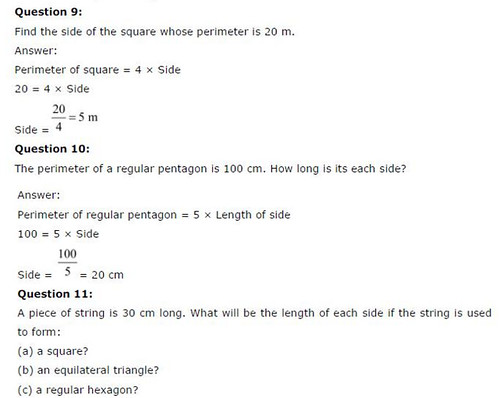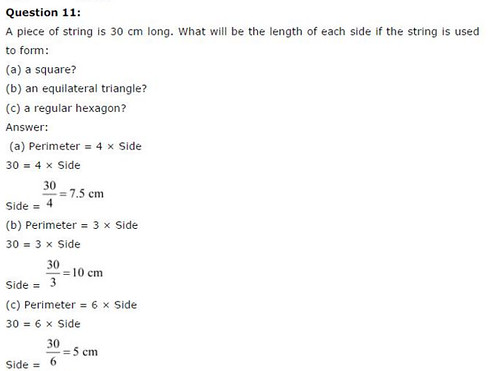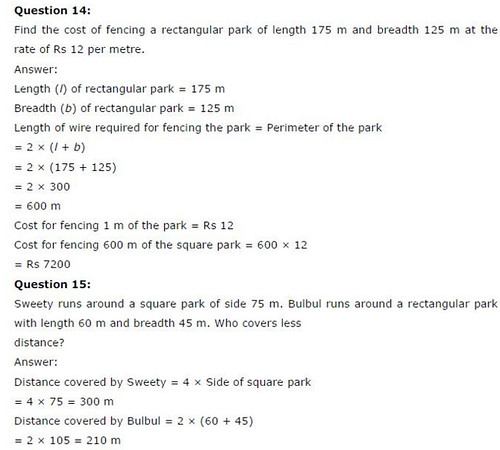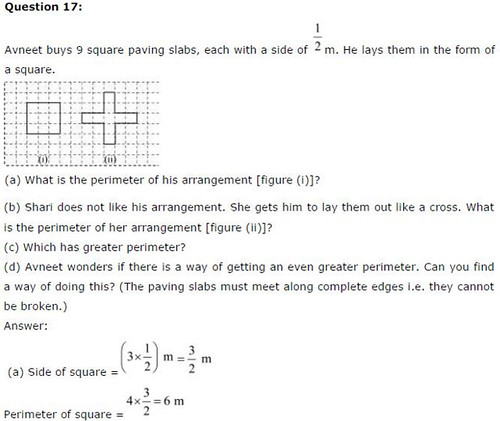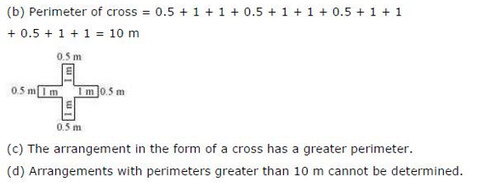## SabDekho

The Complete Educational Website